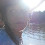## Monday, March 30, 2015

### 33 77 | The Golden Gate Bridge and Recent Symbolism

The bridge has some numbers on it, starting with its '33 construction beginnings and 4200-ft length, emphasis on '42'.  These coordinates above are also something else...
• The = 2+8+5 = 15
• Golden = 7+6+3+4+5+5 = 30
• Gate = 7+1+2+5 = 15
• Bridge = 2+9+9+4+7+5 = 36
• Golden Gate Bridge = 81
• The Golden Gate Bridge = 96
• The = 20+8+5 = 33
• Golden = 7+15+12+4+5+14 = 57
• Gate = 7+1+20+5 = 33
• Bridge = 2+18+9+4+7+5 = 45
• Golden Gate Bridge = 135
• The Golden Gate Bridge = 168
• ***Marin = 13+1+18+9+14 = 55
• ***County = 3+15+21+14+20+25 = 98
• ******Marin County = 153 (A reflection of Golden Gate Bridge)(A number of much importance and pertaining to the Vesica Piscis and other spiritual symbolism)
The bridge opened May 27, the 147th day of the year, 77-years ago.  It took 4 years, 4 months and 22 days to complete.

1.2.